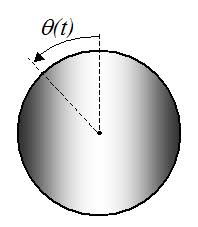How to Calculate and Solve for Superelevation, Guage of Track, Velocity and Radius of a Body in Circular Path Motion | The Calculator EncyclopediaThe image above represents a body in superelevation.

To compute for the Superelevation, four essential parameters are needed and these parameters are Gauge of the track (G), velocity of the body (v), radius of the curve (r) and acceleration due to gravity (g).

The formula for calculating the circular path motion:

S = Gv² / gr

Where:
S = Superelevation
G = Gauge of the track
v = velocity of the body
r = radius of the curve
g = acceleration due to gravity

Let’s solve an example;
Find the circular path motion when Gauge of the track is 14, velocity of the track is 47, radius of the curve is 21 and acceleration due to gravity is 9.8.

This implies that;
G = Gauge of the track = 14
v = velocity of the body = 47
r = radius of the curve = 21
g = acceleration due to gravity = 9.8

S = Gv² / gr
S = 14 x 47² / 9.8 x 21
S = 14 x 2209 / 205.8
S = 30926 / 205.8
S = 150.27

Therefore, the superelevation is 150.27 m.

Calculating the Gauge of the track when Superelevation, Velocity of the body, Radius of the curve and Acceleration due to gravity.

G = Sgr / v2

Where;
G = Gauge of the track
S = Superelevation
v = velocity of the body
r = radius of the curve
g = acceleration due to gravity

Let’s solve an example;
With a superelevation of 180, velocity of the body is 32, radius of the curve is 12 and acceleration due to gravity as 9.8. Find the gauge of the track?

This implies that;
S = Superelevation = 180
v = velocity of the body = 32
r = radius of the curve = 12
g = acceleration due to gravity = 9.8

G = Sgr / v2
G = 180 x 12 x 9.8 / 322
G = 21168 / 1024
G = 20.67

Therefore, the gauge of the track is 20.67.

Calculating the Velocity of the body when Superelevation, Gauge of the track, Radius of the curve and Acceleration due to gravity.

v = √Sgr / G

Where;
v = velocity of the body
S = Superelevation
G = Gauge of the track
r = radius of the curve
g = acceleration due to gravity

Let’s solve an example;
With a superelevation of 120, gauge of the track is 28, radius of the curve is 7 and acceleration due to gravity as 9.8. Find the velocity of the body?

This implies that;
S = Superelevation = 120
G = gauge of the track = 28
r = radius of the curve = 7
g = acceleration due to gravity = 9.8

v = √Sgr / G
v = √120 x 9.8 x 7 / 28
v = √8232 / 28
v = √294
v = 17.146

Therefore, the velocity of the body is 17.146.